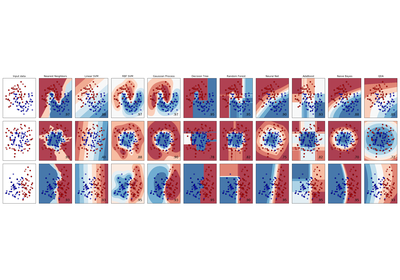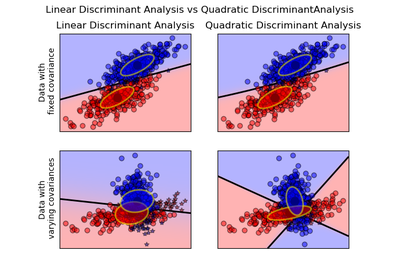# `sklearn.discriminant_analysis`.QuadraticDiscriminantAnalysis¶

class `sklearn.discriminant_analysis.``QuadraticDiscriminantAnalysis`(priors=None, reg_param=0.0, store_covariance=False, tol=0.0001, store_covariances=None)[source]

A classifier with a quadratic decision boundary, generated by fitting class conditional densities to the data and using Bayes’ rule.

The model fits a Gaussian density to each class.

Read more in the User Guide.

Parameters: priors : array, optional, shape = [n_classes] Priors on classes reg_param : float, optional Regularizes the covariance estimate as `(1-reg_param)*Sigma + reg_param*np.eye(n_features)` store_covariance : boolean If True the covariance matrices are computed and stored in the self.covariance_ attribute. New in version 0.17. tol : float, optional, default 1.0e-4 Threshold used for rank estimation. New in version 0.17. covariance_ : list of array-like, shape = [n_features, n_features] Covariance matrices of each class. means_ : array-like, shape = [n_classes, n_features] Class means. priors_ : array-like, shape = [n_classes] Class priors (sum to 1). rotations_ : list of arrays For each class k an array of shape [n_features, n_k], with `n_k = min(n_features, number of elements in class k)` It is the rotation of the Gaussian distribution, i.e. its principal axis. scalings_ : list of arrays For each class k an array of shape [n_k]. It contains the scaling of the Gaussian distributions along its principal axes, i.e. the variance in the rotated coordinate system.

Examples

```>>> from sklearn.discriminant_analysis import QuadraticDiscriminantAnalysis
>>> import numpy as np
>>> X = np.array([[-1, -1], [-2, -1], [-3, -2], [1, 1], [2, 1], [3, 2]])
>>> y = np.array([1, 1, 1, 2, 2, 2])
>>> clf.fit(X, y)
...
store_covariance=False,
store_covariances=None, tol=0.0001)
>>> print(clf.predict([[-0.8, -1]]))

```

Methods

 `decision_function`(X) Apply decision function to an array of samples. `fit`(X, y) Fit the model according to the given training data and parameters. `get_params`([deep]) Get parameters for this estimator. `predict`(X) Perform classification on an array of test vectors X. `predict_log_proba`(X) Return posterior probabilities of classification. `predict_proba`(X) Return posterior probabilities of classification. `score`(X, y[, sample_weight]) Returns the mean accuracy on the given test data and labels. `set_params`(**params) Set the parameters of this estimator.
`__init__`(priors=None, reg_param=0.0, store_covariance=False, tol=0.0001, store_covariances=None)[source]
`covariances_`

DEPRECATED: Attribute covariances_ was deprecated in version 0.19 and will be removed in 0.21. Use covariance_ instead

`decision_function`(X)[source]

Apply decision function to an array of samples.

Parameters: X : array-like, shape = [n_samples, n_features] Array of samples (test vectors). C : array, shape = [n_samples, n_classes] or [n_samples,] Decision function values related to each class, per sample. In the two-class case, the shape is [n_samples,], giving the log likelihood ratio of the positive class.
`fit`(X, y)[source]

Fit the model according to the given training data and parameters.

Changed in version 0.19: `store_covariances` has been moved to main constructor as `store_covariance`

Changed in version 0.19: `tol` has been moved to main constructor.

Parameters: X : array-like, shape = [n_samples, n_features] Training vector, where n_samples is the number of samples and n_features is the number of features. y : array, shape = [n_samples] Target values (integers)
`get_params`(deep=True)[source]

Get parameters for this estimator.

Parameters: deep : boolean, optional If True, will return the parameters for this estimator and contained subobjects that are estimators. params : mapping of string to any Parameter names mapped to their values.
`predict`(X)[source]

Perform classification on an array of test vectors X.

The predicted class C for each sample in X is returned.

Parameters: X : array-like, shape = [n_samples, n_features] C : array, shape = [n_samples]
`predict_log_proba`(X)[source]

Return posterior probabilities of classification.

Parameters: X : array-like, shape = [n_samples, n_features] Array of samples/test vectors. C : array, shape = [n_samples, n_classes] Posterior log-probabilities of classification per class.
`predict_proba`(X)[source]

Return posterior probabilities of classification.

Parameters: X : array-like, shape = [n_samples, n_features] Array of samples/test vectors. C : array, shape = [n_samples, n_classes] Posterior probabilities of classification per class.
`score`(X, y, sample_weight=None)[source]

Returns the mean accuracy on the given test data and labels.

In multi-label classification, this is the subset accuracy which is a harsh metric since you require for each sample that each label set be correctly predicted.

Parameters: X : array-like, shape = (n_samples, n_features) Test samples. y : array-like, shape = (n_samples) or (n_samples, n_outputs) True labels for X. sample_weight : array-like, shape = [n_samples], optional Sample weights. score : float Mean accuracy of self.predict(X) wrt. y.
`set_params`(**params)[source]

Set the parameters of this estimator.

The method works on simple estimators as well as on nested objects (such as pipelines). The latter have parameters of the form `<component>__<parameter>` so that it’s possible to update each component of a nested object.

Returns: self :

## Examples using `sklearn.discriminant_analysis.QuadraticDiscriminantAnalysis`¶Classifier comparisonLinear and Quadratic Discriminant Analysis with covariance ellipsoid